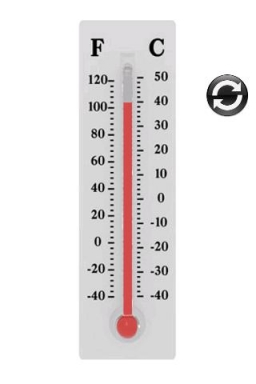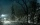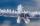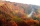# The temperature 16

The temperature at 6 am was 21 degrees Fahrenheit by noon, and the temperature dropped 32 degrees Fahrenheit. What was the temperature at noon?

t2 =  -11 °F

### Step-by-step explanation:Did you find an error or inaccuracy? Feel free to write us. Thank you!## Related math problems and questions:

• Change in temperatureStarting temperature is 21°C, the highest temperature is 32°C. What is the change in temperature?
• WeatherWeather. At 5:00 am, the temperature was 28°C, and at noontime, it rose by 9°C. By 4:00 pm, it dropped 3°C, and by nighttime, it was 4°C less. What is the room temperature this time?
• NoontimeIn the morning temperature was 110°F. By noontime, it has gone up by 15°F. What was the noon temperature? (Give your answer in °F)
• At noon 2At noon, the temperature was 4 degrees fahrenheit. At 6pm, it was 10 degrees colder. What was the temperature at 6pm?
• OpheliaOphelia recorded the temperature of a cold store every two hours. 1. At 6 am, it was -4°C, and at 8 am, it was -1°C. By how much did the temperature rise? 2. The temperature went up by 5°C in the next two hours. .What was the temperature at 10 am?
• Outdoor temperatureThe outdoor temperature was 8 degrees at midnight. The temperature declined 5 degrees during each of the next 3 hours. What was the temperature at 3am?
• At midmorningThe temperature at midmorning was 31°C. Early in the evening, the temperature dropped by 5°C. What was the resulting temperature?
• The temperature 4The temperature was freezing, then it doubled, then it dropped 10 degrees, and then it increased by 40 degrees. The temperature is now 16 degrees. What was the starting temperature?
• LaboratoryMang Elio went to his laboratory at 7:00am And recorded that the temperature was 11.07°C. At lunchtime, the temperature rose by 27.9°C but dropped by 8.05°C at 5:00 PM. What was the temperature at 5:00 PM?
• The temperature 3The temperature is -12 degrees Fahrenheit. During the following 5 hours, the temperature decrease by 23 degrees Fahrenheit. What is the weather at 11 pm?
• Outside temperatureThe temperature outside was 57 degree Fahrenheit. During the next few hours it decreased by 18 degrees and then increased by 23 degrees. Find new temperature.
• Midnight 2It was 8 degrees at nightfall. The temperature dropped 10 by midnight. What was the temperature at midnight?
• At noonAt noon today it was 6°C outside, but it has increased 4°C since then. What is the temperature outside now?
• The freezer1. The temperature inside a freezer is minus 23 degree Celsius. The temperature falls by a further 12 degree degree Celsius. What is the new temperature? 2. What is the difference between temperatures of 12 degree Celsius and 210 degree Celsius?
• Temperature rise and fallOn Friday, the temperature was 82°F. The temperature changed by –2°F on Saturday, and then it changed by 5°F on Sunday. What was the temperature on Sunday? How did the temperature change? Note we consider the mathematical problem formulated in this way to
• Change per hourOn Sunday, the temperature was 63 degrees at 2 pm. The temperature was then 72 degrees at 5 pm. What is the rate of change per hour?
• The bathDad poured 50 liters of water at 60°C into the tub. How much must cold water be added at 10°C to obtain a bath that has a temperature of 40°C?